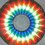# Another geometry problem? Noooo.

Prove that a right angled triangle with all integer sides has an area that is divisible by 6.

Solution:

Let's look at the fundamental Pythagorean triplets instead of looking at their similar triangles. So we have the sides: $(m^2+n^2), 2mn, (m^2-n^2)$ , where m and n are two coprime natural numbers.

The area becomes: $(m-n)(m+n)mn.$ As m and n are coprime, if they are of the same parity, then they both have to be odd and hence, a factor of 4 and hence 2, is established in side $m^2-n^2.$ If the parity is even for either one of them, then a factor of 2 is established in the product mn. Now we need to look for a factor of 3 in order to prove the question.

If either one of m and n is a multiple of 3, then there's no question about it since the factor gets established in the product mn.

Otherwise, W.L.O.G*, let m and n have the configuration:

• 3k+1, 3l+1.
• 3k+1, 3l+2.
• 3k+2, 3k+2.

respectively

We can immediately see that in the first and last cases, the difference of m and n creates a factor of three in the area. In the second case, the sum creates a factor of three. Hence the area is going to be divisible by 6.

Q.E.D

*= Without Loss Of Generality.

If you want to impress your friends and relatives at a dinner party or whatever, you can ask them to do this:

• Think of any two numbers.
• Add those two numbers, subtract those two numbers and multiply the two numbers.
• Now write down your prediction on a piece of paper that says the product of the 3 resultant numbers is going to be a multiple of 3.
• They will think that you got lucky. So let them have a go at it again and again and again.... till they give up and ask you how to do it. Explain the method and look like a genius.Note by Vishnu C
6 years, 2 months ago

This discussion board is a place to discuss our Daily Challenges and the math and science related to those challenges. Explanations are more than just a solution — they should explain the steps and thinking strategies that you used to obtain the solution. Comments should further the discussion of math and science.

When posting on Brilliant:

• Use the emojis to react to an explanation, whether you're congratulating a job well done , or just really confused .
• Ask specific questions about the challenge or the steps in somebody's explanation. Well-posed questions can add a lot to the discussion, but posting "I don't understand!" doesn't help anyone.
• Try to contribute something new to the discussion, whether it is an extension, generalization or other idea related to the challenge.

MarkdownAppears as
*italics* or _italics_ italics
**bold** or __bold__ bold
- bulleted- list
• bulleted
• list
1. numbered2. list
1. numbered
2. list
Note: you must add a full line of space before and after lists for them to show up correctly
paragraph 1paragraph 2

paragraph 1

paragraph 2

[example link](https://brilliant.org)example link
> This is a quote
This is a quote
    # I indented these lines
# 4 spaces, and now they show
# up as a code block.

print "hello world"
# I indented these lines
# 4 spaces, and now they show
# up as a code block.

print "hello world"
MathAppears as
Remember to wrap math in $$ ... $$ or $ ... $ to ensure proper formatting.
2 \times 3 $2 \times 3$
2^{34} $2^{34}$
a_{i-1} $a_{i-1}$
\frac{2}{3} $\frac{2}{3}$
\sqrt{2} $\sqrt{2}$
\sum_{i=1}^3 $\sum_{i=1}^3$
\sin \theta $\sin \theta$
\boxed{123} $\boxed{123}$